# Momocs speed dating

## Preliminaries

### Abstract

Momocs aims to provide a complete and convenient toolkit for morphometrics. It is intended for scientists interested in describing quantitatively the shape, and its (co)variations, of the objects they study.

In the last decade, R has become the open-source lingua franca for statistics, and morphometrics known its so-called “revolution”. Nevertheless, morphometric analyses still have to be carried out using various software packages either dedicated to a particular morphometric and/or for which source code is mostly unavailable and/or copyrighted. Moreover, most of existing software packages cannot be extended and their bugs are hard to detect and thus correct. This situation is detrimental to morphometrics: time is wasted, analyses are restricted to available methods, and last but not least, are poorly reproducible. This impedes collaborative effort both in software development and in morphometric studies.

By gathering the common morphometric approaches in an open-source environment and welcoming contributions, Momocs is an attempt to solve this twofold problem.

Momocs hinges on the core functions published in the must-have Morphometrics with R by Julien Claude (2008), but has been further extended to allow other shape description systems. So far, configurations of landmarks, outlines and open outline analyses, along with some facilities for traditional morphometrics are implemented.

Prior to analysis, Momocs can be used to acquire and manipulate data or to import/export from/to other formats. Momocs also has the facility for a wide range of multivariate analyses and production of the companion graphics. Thus a researcher will find that just a few lines of code will provide initial results, but the methods implemented can be finely tuned and extended according to the user’s needs.

• See Momocs features.
• If you use it, cite it: `citation("Momocs")`.
• This citation refers to an obsolete version of Momocs, only handling outline analyses. The next companion and seminal paper is submitted.

### Survival tips

• This vignette introduces Momocs; more specific help can be find in function’s help files, for instance `?efourier`.
• There is a much nicer onlineversion of this manual that can be accessed from the console with, e.g. `Momocs_help("efourier")`.
• Feel free to contribute to Momocs through GitHub: report issues, ask for new features, share data and methods, correct typos, write better vignettes, helpfiles, or whatever pleases you. If you have never heard of GitHub, that’s definitely worth a look.
• Feel free to drop me a line, should you need a hand or would like to collaborate with me: `bonhomme.vincent@gmail.com`.

### Get, install and use it

First, of all, let’s download the last version of Momocs. You will need to install the `devtools` package to get it from my GitHub repository :

``````install.packages("devtools")
devtools::install_github("vbonhomme/Momocs")``````

The typical `install_packages("Momocs")` will get you the last CRAN version of Momocs, but the GitHub version is preferred as Momocs is still under active development.

We can start using Momocs, as long as it has been loaded using:

``library(Momocs)``

## Design

Keywords used all accross Momocs are introduced here in bold.

Morphometrics is the ugly job of turning beautiful shapes into quantitative variables. Just kidding, that’s pretty exciting.

A shape is defined as a collection of `(x; y)` coordinates. No 3D yet but different families can be handled: outlines, here in a first-quarter moon ; open outlines, here is the sterile valve of an olive stone; configuration of landmarks; here, hologous points from a mosquito wing.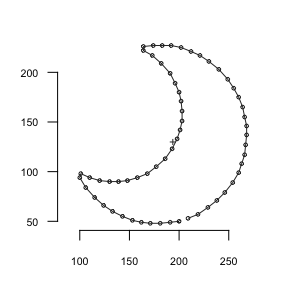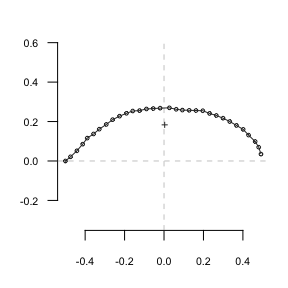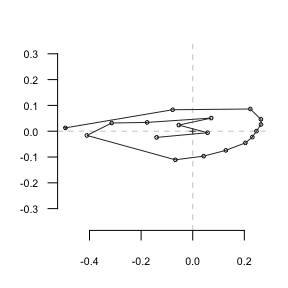They are all single shapes defined by a matrix of `(x; y)` coordinates; here are the first points of the moon:

``shapes %>% head()``
``````##      [,1] [,2]
## [1,]  200   50
## [2,]  199   49
## [3,]  198   49
## [4,]  197   50
## [5,]  196   50
## [6,]  195   49``````

A few dozens of operations on single shapes are implemented such as: plotting, centering, calculating areas, etc. These 70+ operations can be accessed with `apropos("coo_")`. But working on single shapes is quite boring.

Shapes can be organized into a collection of coordinates: a `Coo` object that carries:

• a component named `\$coo`, a `list` of shapes (as `matrix`.ces);
• most of the time, a component named `\$fac`, a `data.frame` to store covariates, either `factor`s or `numeric`s;
• possibly, other components of interest.

One can do several things with a `Coo` object: visualize it, apply morphometric operations, handle the data it contains, but in the end, a __ morphometric method__ will turn coordinates into coefficients.

Such morphometric operation on coordinates produce a collection of coefficients: a `Coe` object that carries:

• a component named `\$coe`, a `matrix` of coefficients;
• if present in `Coo`, `\$fac` is inherited;
• possibly, other components of interest.

This can be summarized as follows:

`Coo` + Morphometric method = `Coe`
`(x; y)` coordinates + appropriate method = quantitative variables

`Coo` objects are collections of coordinates that become `Coe` objects when an appropriate morphometric method is applied on them.

Some operations on `Coo`/`Coe` are generic in that they do not depend of the nature of the shape. For instance, centering a configuration of landmarks or an outline, or calculating their centroid size is, mathematically, the same generic operation. But some operations on shapes are specific to a peculiar family. For instance, calculating elliptical Fourier transforms on a configuration of landmarks would make no sense.

Momocs implement this desirable behavior and defines classes and subclasses, as S3 objects.

`Coo` Morphometrics methods `Coe`
`OutCoo` (outlines) `efourier`, `rfourier`, `sfourier`, `tfourier` `OutCoe`
`OpnCoo` (open outlines) `npoly`, `opoly`, `dfourier` `OpnCoe`
`LdkCoo` (configuration of landmarks) `fgProcrustes`, `slide` `LdkCoe`

You can see this table as a scheme there.

In other words:

• any collection of shapes belongs to (pick one) `{OutCoo, OpnCoo, LdkCoo}` and is also a `Coo` object;
• generic and specific methods can be applied to it
• a collection of coefficients is obtain and belongs to (pick one) `{OutCoe, OpnCoe, LdkCoe}` and is also a `Coe` object.

Finally, generic and specific operations can be applied to the `Coe` objects, chiefly multivariate methods, capitalicized: `PCA`, `LDA`, `CLUST`, `MANOVA`(and MANCOVA), `MSHAPES`, `KMEANS`, etc.

Overall, Momocs implements a simple and consistent grammar that is detailed below. Also, if you’re familiar with modern R and the Hadley-verse, you should feel home as `ggplot2` graphics, `dplyr` verbs and `magrittr` pipes are implemented.

## Single shapes

Let’s load one of the Momocs datasets, some various outlines (an `Out` object):

``````shapes                    # prints a brief summary
panel(shapes, names=TRUE) # base graphics``````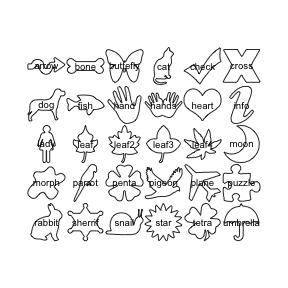``panel2(shapes)            # ggplot2 graphics``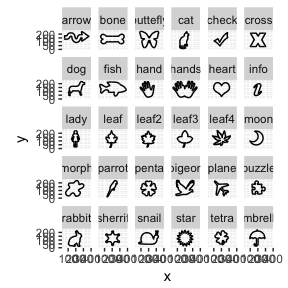``````## An Out object with:
##  - \$coo: 30 outlines (836 +/- 255 coordinates, all closed)
##  - \$fac: No classifier defined in \$fac``````

`shapes` is one of the datasets bundled with Momocs. It’s (“lazy”) loaded in memory as soon as you call it, no need for `data(shapes)`. To see all Momocs’ datasets, try `data(package="Momocs")`. These datasets are all `Coo` objects (try `class(bot)`), ie collection of shapes.

One can do many things on a `Coo` object, as above, e.g. printing a summary of it (just by typing its name in the console), plotting a family picture with `panel` or `panel2`. Note the `2` that refers to a `ggplot2` variant of a given plot.

So far, we’re interested in single shapes so let’s extract the 4th shape from `shapes`, using the traditional syntax. We plot it with `coo_plot` that comes with several options for plotting all families of shapes.

``````shp <- shapes
coo_plot(shp)``````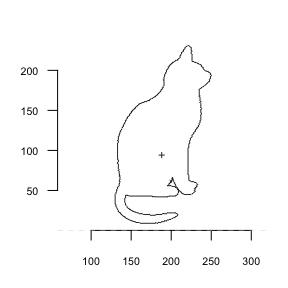``````# coo_plot is the base plotter for shapes
# but it can be finely customized, see ?coo_plot
coo_plot(shp, col="grey80", border=NA, centroid=FALSE, main="Meow")``````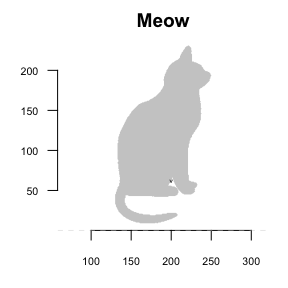Let’s now do some basic operations on this shape. They all named `coo_*` and you can have the full list with `apropos("coo_")`. `coo_*` family encompasses:

• geometric operations (such as centering, scaling, etc.)
• plotting functions
• scalar descriptors of shape (such as area, perimeter, circularity, rectilinearity, etc.)
• various other operations on a single shape.
``coo_plot(coo_center(shp), main="centered Meow")``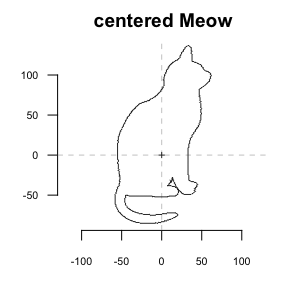``coo_plot(coo_sample(shp, 64), points=TRUE, pch=20, main="64-pts Meow")``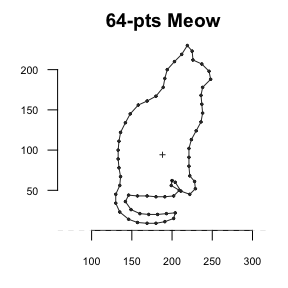Momocs makes use of maggritr’s pipe operators. A nice introduction can be found there. `magrittr` requires a (very small) cerebral gymnastics at the beginning but the benefits are huge, for defining moprhometric pipelines in Momocs but also for R as a whole. It makes things clearer, it: saves typing; reduces intermediate variable assignation; reads from left to right; substantiates the pipe we (should) have in mind. `magrittr`’s pipes are already loaded with Momocs.

``shapes %>% coo_smooth(5) %>% coo_sample(64) %>% coo_scale() %>% coo_plot()``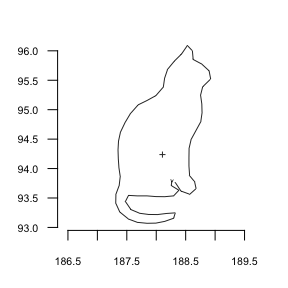``````# pipes can be turned into custom function
cs64 <- function(x) x %>% coo_sample(64) %>% coo_scale() %>% coo_center()
shapes %>% cs64 %>% coo_plot() # note the axes``````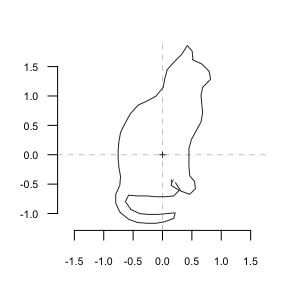The most familiar operation can directly be applied on `Coo` objects:

``````bot %>%
coo_center %>% coo_scale %>%
coo_alignxax() %>% coo_slidedirection("N") %T>%
print() %>% stack()``````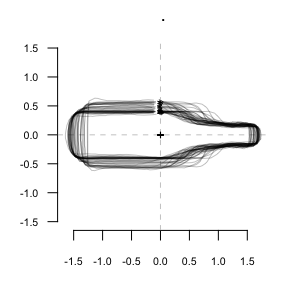``````## An Out object with:
##  - \$coo: 40 outlines (162 +/- 21 coordinates, all unclosed)
##  - \$fac: 1 classifier:
##      'type' (factor 2): beer, whisky.``````

## Morphometrics

### Outline analysis

A word about data import: you can extract outlines from a list of black masks over a white background, as `.jpg` images with `import_jpg`. Have a look to helpfiles (`import_jpg` and `import_jpg1`) for more details. Here we do not bother with import since we will use the `bot`tles outlines dataset bundled with Momocs.

``````data(bot)
bot
panel(bot, fac="type", names=TRUE)``````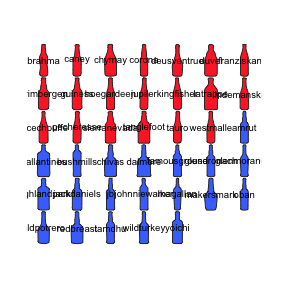``stack(bot)``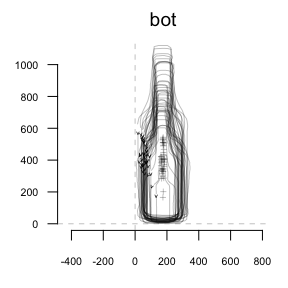``````## An Out object with:
##  - \$coo: 40 outlines (162 +/- 21 coordinates, all unclosed)
##  - \$fac: 1 classifier:
##      'type' (factor 2): beer, whisky.``````

Here we will illustrate outline analysis with some elliptical Fourier transforms (but the less used - and tested - radii variation Fourier transforms, its variant used by Renaud et al., and tangent angle Fourier transforms are also implemented with `rfourier`, `sfourier` and `tfourier` respectively).

The idea behind elliptical Fourier transforms is to fit the x and y coordinates separately, that is the blue and red curves below:

``coo_oscillo(bot, "efourier")``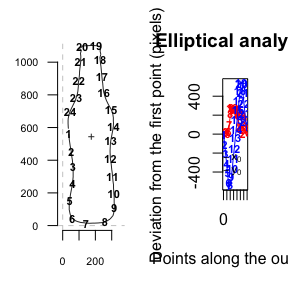Graphically, this is equivalent to fitting Ptolemaic ellipses on the plane:

``Ptolemy(bot)``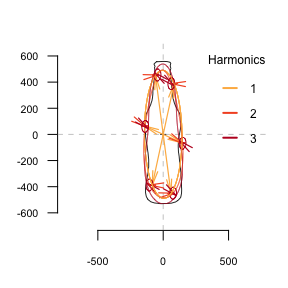Let’s calibrate the number of harmonics required. More details can be found in their respective help files.

``calibrate_harmonicpower(bot)``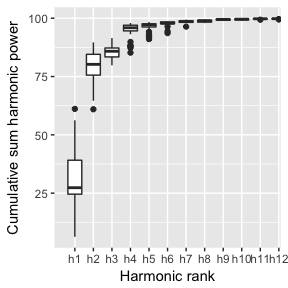``calibrate_deviations(bot)``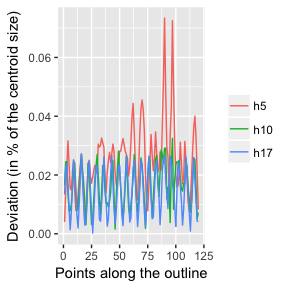``calibrate_reconstructions(bot)``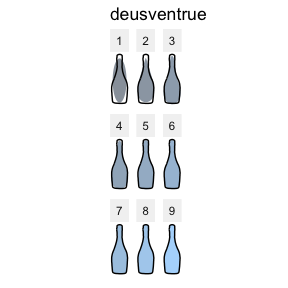``````##   90%   95%   99% 99.9%
##     5     5    10    17``````

Here, 10 harmonics gather 99% of the harmonic power. If you’re happy with this criterium, you can even omit `nb.h` in `efourier`: that’s the default parameter, returned with a message.

``````bot.f <- efourier(bot, nb.h=10)
bot.f``````
``````## An OutCoe object [ elliptical Fourier analysis ]
## --------------------
##  - \$coe: 40 outlines described, 10 harmonics
##  - \$fac: 1 classifier:
##      'type' (factor 2): beer, whisky.``````

`bot.f` is a `Coe` object (and even an `OutCoe`), you have have a look to the help files to go deeper into Momocs classes.

Let’s have a look to the amplitude of fitted coefficients.

``hist(bot.f, drop=0)``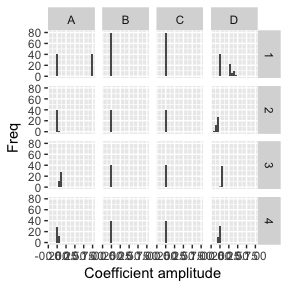``boxplot(bot.f, drop=1)``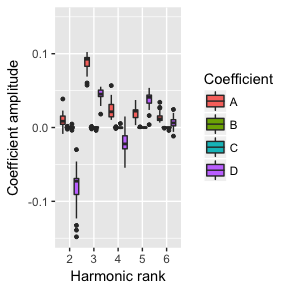Now, we can calculate a PCA on the `Coe` object and plot it, along with morphospaces, calculated on the fly.

``````bot.p <- PCA(bot.f)
class(bot.p)        # a PCA object, let's plot it
plot(bot.p)``````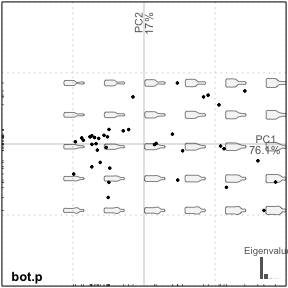``##  "PCA"    "prcomp"``

Amazing but we will do much better afterwards.

The question of normalization in elliptical Fourier transforms is central. Normalization can either be done beforehand, with geometric operations, or afterhand, directly on the matrix of Fourier coefficients and consuming the first harmonic. In brief, I’m not a big fan of the “use the first harmonics and see what happens” strategy, as some biases can be introduced (and actually quite hard to detect), particularly on rounded/ellipsoid shapes. More can be found in `?efourier`.

Here is an example of such bias from the `molars` dataset generously shared by Cornu and Detroit.

``````# raw molars dataset
stack(molars, title = "Non-aligned molars")``````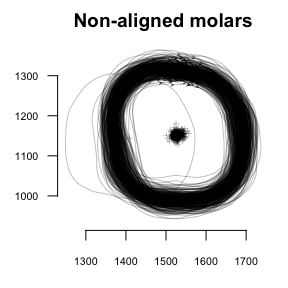``````# Procrustes-aligned and slided molars
mol.al <- fgProcrustes(molars, tol = 1e-4) %>% coo_slidedirection("W")
stack(mol.al, title="Aligned molars")``````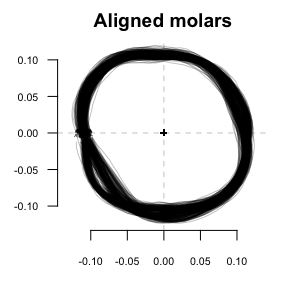``````# Now compare PCA and morphospace using the 1st harmonic alignment
molars %>% efourier(norm=TRUE) %>% PCA() %>% plot("type")``````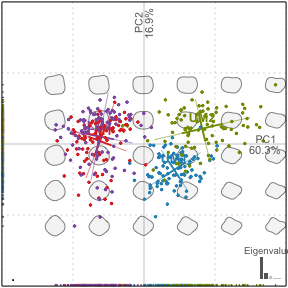``````# and the a priori normalization
molars %>% efourier(norm=FALSE) %>% PCA() %>% plot("type")``````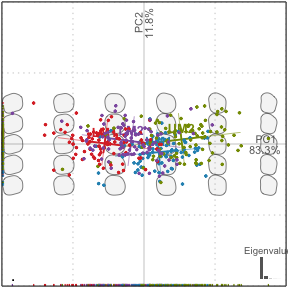Finally, you can drop some harmonics with `rm_harm`. And methods that removes the bilateral (a)symmetry are implemented: `rm_asym` and `rm_sym`, while `symmetry` calculates some related indices.

### Open outlines

Open outlines are curves. Methods actually implemented are:

• `npoly` that fit natural polynomials;
• `opoly` that fit orthogonal (also called Legendre’s) polynomials;
• `dfourier` for the discrete cosine transform.

Note that `opoly` and `npoly` can only be used on simple curves, curves that have at most one `y` for any `x` coordinates, at least under a given orientation. `dfourier` can fit complex curves, curves “that back on their feets”.

Here, we will work on the fertile valves of olive stones, a (very partial) dataset provided by my colleagues Terral, Ivorra, and others.

They have two orthogonal views (a lateral and a dorsal view). See the paper cited in `?olea` for more details. Let’s explore it a bit:

``````olea
stack(olea, fac="view")     # already aligned \o/``````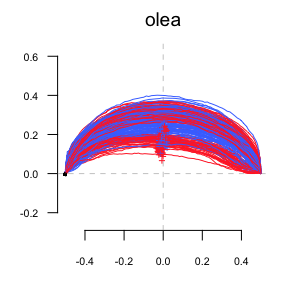``panel(olea, names="ind")    # another family picture``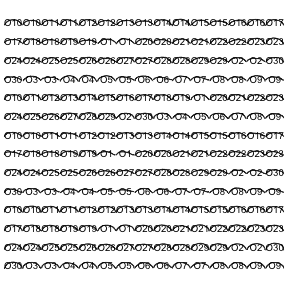``````## An Opn object with:
##  - \$coo: 210 open outlines (99 +/- 4 coordinates)
##  - \$fac: 4 classifiers:
##      'var' (factor 4): Aglan, Cypre, MouBo1, PicMa.
##      'domes' (factor 2): cult, wild.
##      'view' (factor 2): VD, VL.
##      'ind' (factor 30): O1, O10, O11, O12, O13, O14, O15, O16, O17, O18, O19 ... + 19 more.``````

Now, we gonna calculate `opoly` on it and plot the result of the PCA. Notice how consistent is the grammar and the objects obtained:

``````op <- opoly(olea)           # orthogonal polynomials
class(op)                   # an OpnCoe, but also a Coe
op.p <- PCA(op)             # we calculate a PCA on it
class(op.p)                 # a PCA object
plot(PCA(op), ~domes+var)   # notice the formula interface to combine factors``````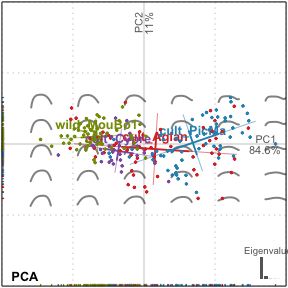``````##  "OpnCoe" "Coe"
##  "PCA"    "prcomp"``````

But this is perfectly wrong! We merged the two views are if they were different individuals. Momocs can first `chop` or `filter` the whole dataset to separate the two views, do morphometrics on them, and `combine` them afterwards.

``````table(olea, "view", "var")
# we drop 'Cypre' since there is no VL for 'Cypre' var
olea %>% filter(var != "Cypre") %>%
# split, do morphometrics, combine
chop(view) %>% lapply(opoly) %>% combine() %T>%
# we print the OpnCoe object, then resume to the pipe
print() %>%
# note the two views in the morphospace
PCA() %>% plot("var")``````